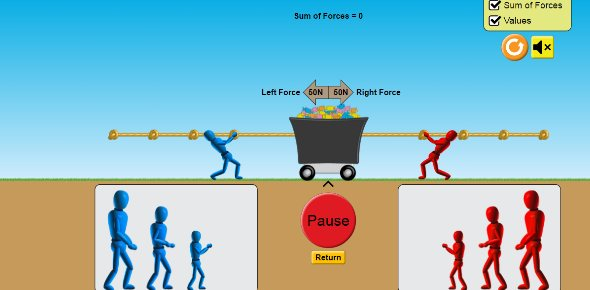# Force And Friction!

7 Questions | Total Attempts: 202SettingsHey Milz, here is a short quiz for you xx

Related Topics
• 1.
What is a force?
• A.

Like a tree

• B.

A force that is magnetic

• C.

A push or pull action that affects an object

• D.

A push or pull force that affects just humans

• 2.
Name he four forces
• 3.
Give a breif explanation of what friction is
• 4.
Name ONE way friction can be reduced
• 5.
What can force do?
• A.

Change an object in shape or motion

• B.

Change the colour of an object

• C.

Make an obect smaller or larger

• 6.
What can force be measured by?
• A.

A forceometer

• B.

A forceogrameter

• C.

A force meter

• D.

A force measurer

• 7.
When a rubber band is pulled what happens to it? Why?
• A.

It changes motion and shape because of the elastic

• B.

It changes shape but not motion because of force

• C.

It changes shape but not motion because of gravity